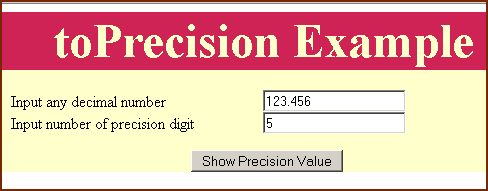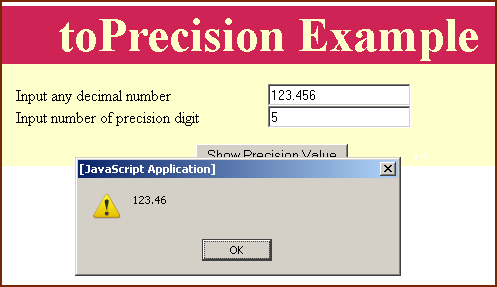Tutorials

JavaScript toPrecision method

In JavaScript, toPrecision() method is used to convert a number into the number with the number of digits as provided for the precision.

In JavaScript, toPrecision() method is used to convert a number into the number with the number of digits as provided for the precision.

JavaScript toPrecision method

In JavaScript, toPrecision() method is used to convert a number into the number with the number of digits as provided for the precision.

Syntax:

 number_object.toPrevent( digits);

Where number_object is the number at which we have to apply method toPrecision() and digits are the number of digits for the number value.  If the digits is greater than the number of digits in the number_object then it automatically adds "0"(zero) to show the number value in the provided number of digits. For example if we have a number like 123.456 and we have provided the number of digits for precision 7 then it would convert that number like 123.4560. If the digits is less than the number of digits in the number_object then it will round the number_object.

Description of code:

To illustrate the use of toPrecision() method we have created a simple page containing two text boxes. First text box is used to take the number value which is to be converted and second text box is used for number of digits for precision. We also have created a button "Show Precision Value" which calls a function callToPrecision() when clicked.

 function callToPrecision(){ var val= new Number(document.getElementById( "txt").value); var digits = document.getElementById( "txtprc").value; alert(val.toPrecision(digits)); }

In above lines of code we have declared a variable "val" which holds the number value of the text box and we have taken the text box value by the method document.getElementById("txt").value. In the second line of function we have taken the number of digits into a variable "digits". Now since we have both "number value" and  "number of digits" we can apply toPrecision() method.

Here is the full HTML code as follows :


toPrecision Example

Input any decimal number
Input number of precision digit

Output :

Suppose we enter number "123.456" and number of precision digit 5 i.e. we have to convert number 123.456 into a 5 digit number.If we apply toPrecision() method over it then it will result a converted 5-digit number after rounding 123.46 as shown in the output.Now if we insert number of digits less than the digits present before the decimal point then it automatically converts the number in the exponential format as shown in the output.As you can see from the following output that if the number of digits are less in the number value in comparison to the provided number of digits for precision then toPrecision() method automatically converts the number in the specified digit by adding extra "0" at the end of number after the last decimal number place. For example if we insert a decimal number like "123.456" and number of digits for precision number are "8" then it automatically converts number like 123.45600If the number of precision digits are same as the number of digits before decimal point of input decimal number, then it returns the number without decimal point. For example,